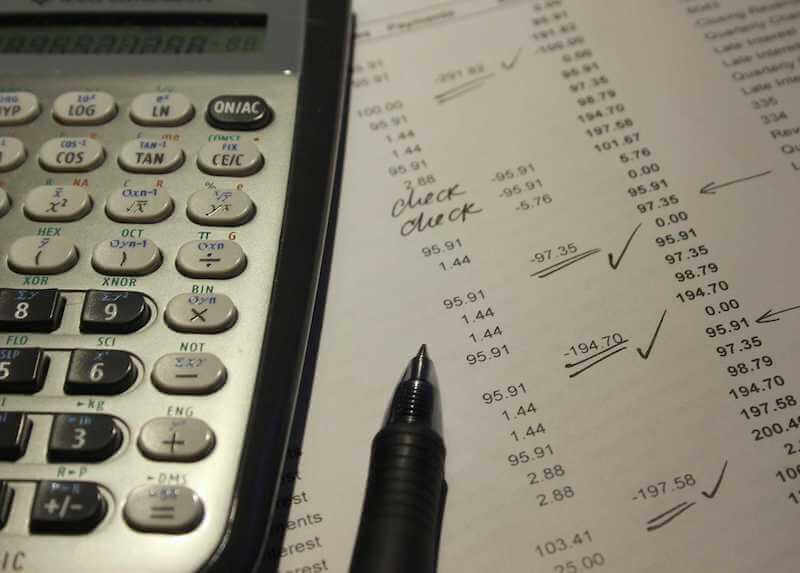This is the formula for calculating cost per mile.

PURCHASE PRICE - PREDICTED SELL OR TRADE PRICE + INTEREST ON LOAN + ESTIMATED MILEAGE = DEPRECIATION COST PER MILE

This is the formula for calculating cost per day.

PURCHASE PRICE - PREDICTED SELL OR TRADE PRICE + INTEREST ON LOAN + YEARS OWNED x 365 or 260 = COST PER DAY

Depreciation Worksheet

COMPARABLE UNITS                             PRICE

____________________                                            _

____________________________+___________________

____________________________+___________________

____________________________+___________________

____________________________+___________________

TOTAL ______________

ESTIMATED SELLING PRICE       =______________

PURCHASE PRICE     _____________

SELLING PRICE       -_____________

INTEREST PAID      +_____________
TOTAL                     =_____________   _______________
MILES UTILIZED      ~_____________~ DAYS 365 OR 260
COST PER MILE     =_____________= _______________(COST PER DAY)
IN DEPRECIATION